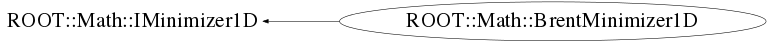# class ROOT::Math::BrentMinimizer1D: private ROOT::Math::IMinimizer1D

```
User class for performing function minimization

It will use the Brent Method for function minimization in a given interval.
First, a grid search is used to bracket the minimum value
with the a step size = (xmax-xmin)/npx. The step size
can be controlled via the SetNpx() function. A default value of npx = 100 is used.
The default value con be changed using the static method SetDefaultNpx.
If the function is unimodal or if its extrema are far apart, setting the fNpx to
a small value speeds the algorithm up many times.
Then, Brent's method is applied on the bracketed interval.
If the Brent method fails to converge the bracketing is repeted on the latest best estimate of the
interval. The procedure is repeted with a maximum value (default =10) which can be set for all
BrentRootFinder classes with the method SetDefaultNSearch

This class is implemented from TF1::GetMinimum.

To use the class, three steps have to be taken:
1. Create the class.
2. Set a function within an interval to look for the minimum.
3. Call the Minimize function with the error parameters.

If another minimization is to be performed, repeat the last two steps.

@ingroup Min1D

```

## Function Members (Methods)

public:
 virtual ~BrentMinimizer1D() ROOT::Math::BrentMinimizer1D BrentMinimizer1D() ROOT::Math::BrentMinimizer1D BrentMinimizer1D(const ROOT::Math::BrentMinimizer1D&) virtual double FValLower() const virtual double FValMinimum() const virtual double FValUpper() const virtual int Iterations() const virtual bool Minimize(int maxIter, double absTol = 1.0E-8, double relTol = 1.0E-10) virtual const char* Name() const ROOT::Math::BrentMinimizer1D& operator=(const ROOT::Math::BrentMinimizer1D&) static void SetDefaultNpx(int npx) static void SetDefaultNSearch(int n) void SetFunction(const ROOT::Math::IGenFunction& f, double xlow, double xup) void SetLogScan(bool on) void SetNpx(int npx) virtual int Status() const virtual double XLower() const virtual double XMinimum() const virtual double XUpper() const

## Data Members

private:
 const ROOT::Math::IGenFunction* fFunction Pointer to the function. bool fLogScan flag to control usage of a log scan int fNIter Number of iterations needed for the last estimation. int fNpx Number of points to bracket minimum with grid (def is 100) int fStatus Status of code of the last estimate double fXMax Upper bound of the search interval double fXMin Lower bound of the search interval. double fXMinimum Position of the stimated minimum.

## Class Charts## Function documentation

``` Default Constructor.
```
virtual ~BrentMinimizer1D()
``` Default Destructor.
```
`{}`
double XMinimum() const
``` Return current estimate of the position of the minimum.
```
`{ return fXMinimum; }`
double XLower() const
``` Return current lower bound of the minimization interval.
```
`{ return fXMin; }`
double XUpper() const
``` Return current upper bound of the minimization interval.
```
`{ return fXMax; }`
double FValMinimum() const
``` Return function value at current estimate of the minimum.
```
double FValLower() const
``` Return function value at current lower bound of the minimization interval.
```
double FValUpper() const
``` Return function value at current upper bound of the minimization interval.
```
bool Minimize(int maxIter, double absTol = 1.0E-8, double relTol = 1.0E-10)
``` Find minimum position iterating until convergence specified by the absolute and relative tolerance or
the maximum number of iteration is reached.
Return true if iterations converged successfully
\@param maxIter maximum number of iterations.
\@param absTol desired absolute error in the minimum position (default 1.E-8)
\@param absTol desired relative error in the minimum position (default = 1.E-10)

```
int Iterations() const
``` Return number of iteration used to find minimum
```
`{ return fNIter; }`
const char * Name() const
``` Return name of minimization algorithm ("BrentMinimizer1D")
```
void SetFunction(const ROOT::Math::IGenFunction& f, double xlow, double xup)
``` Sets function to be minimized.

\@param f Function to be minimized.
\@param xlow Lower bound of the search interval.
\@param xup Upper bound of the search interval.

```
void SetNpx(int npx)
``` Set the number of point used to bracket root using a grid
```
`{ fNpx = npx; }`
void SetLogScan(bool on)
```          Set a log grid scan (default is equidistant bins)
will work only if xlow > 0

```
`{ fLogScan = on; }`
int Status() const
``` Returns status of last estimate. If = 0 is OK
```
`{ return fStatus; }`
void SetDefaultNpx(int npx)
``` static function used to modify the default parameters
set number of default Npx used at construction time (when SetNpx is not called)
Default value is 100

```
void SetDefaultNSearch(int n)
``` set number of  times the bracketing search in combination with is done to find a good interval
Default value is 10

```